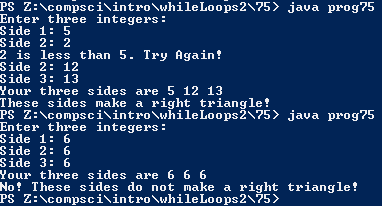# Assignemnt #75, Right Triangle Checker

## Code

```      /// Name: Alex Pinder
/// Period: 5
/// Program Name: Right Triangle Checker
/// File Name: prog75.java
/// Date Complete: 1/27/16

import java.util.Scanner;

public class prog75
{
public static void main( String[] args )
{
Scanner keyboard = new Scanner(System.in);

int a, b, c;

System.out.println( "Enter three integers:" );

do
{
System.out.print( "Side 1: ");
a = keyboard.nextInt();

if ( a <= 0 )
System.out.println( "That doesn't even make sense! Choose numbers that are of absolute value!" );

} while ( a <= 0 );

do
{
System.out.print( "Side 2: " );
b = keyboard.nextInt();

if ( b < a )
System.out.println( b + " is less than " + a + ". Try Again!" );
}   while ( b < a );

do
{
System.out.print( "Side 3: " );
c = keyboard.nextInt();

if ( c < b )
System.out.println( c + " is less than " + b + ". Try Again!" );
}   while ( c < b );

System.out.println( "Your three sides are " + a + " " + b + " " + c );

double triangle = Math.pow( a, 2 ) + Math.pow( b, 2 );

if ( triangle == Math.pow( c, 2 ) )
System.out.println( "These sides make a right triangle!" );
else if ( triangle != Math.pow( c, 2 ) )
System.out.println( "No! These sides do not make a right triangle!" );
}
}
```

### Picture of the output